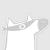British Machine Vision Conference为在英国从事机器视觉、图像处理和模式识别的个人和组织提供了一个国家论坛。其主要目的是：提高机器视觉和模式识别知识、鼓励技术的实际应用、促进研究成果迅速向产业转移、在国内外代表英国机器视觉界官网链接：https://britishmachinevisionassociation.github.io/0+0+0+0+0+0+0+0+0+0+0+0+0+0+0+0+0+0+0+0+0+0+0+0+0+0+0+0+0+0+0+0+0+0+0+0+0+0+0+0+0+0+0+0+0+
Top